International Journal of Theoretical and Applied Mathematics
Volume 3, Issue 2, April 2017, Pages: 70-76

Near Ground Path Loss Prediction for UMTS 2100 MHz Frequency Band Over Propagating Over a Smooth-Earth Terrain

Umesi Cosmos Nnanyerem1, Ozuomba Simeon2, Afolayan J. Jimoh2

1Department of Computer Engineering, Michael Okpara University of Agriculture, Umudike, Nigeria

2Department of Electrical/Electronic and Computer Engineering, University of Uyo, Uyo, Nigeria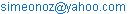(O. Simeon)

Umesi Cosmos Nnanyerem, Ozuomba Simeon, Afolayan J. Jimoh. Near Ground Path Loss Prediction for UMTS 2100 MHz Frequency Band Over Propagating Over a Smooth-Earth Terrain. International Journal of Theoretical and Applied Mathematics. Vol. 3, No. 2, 2017, pp. 70-76.
doi:
10.11648/j.ijtam.20170302.14

Received: October 25, 2016; Accepted: January 17, 2017; Published: February 16, 2017

Abstract: In this paper, path loss prediction for near ground propagation of third generation (3G)-based Universal Mobile Telecommunications System (UMTS) network signal in the 2100 MHz frequency band over a smooth-earth terrain is presented. Particularly, the attenuation due to diffraction is estimated based on ITU-R Recommendation P.526-13 for diffraction over smooth earth. Furthermore, the total pathloss is determined using the Blomquist empirical model which combined free-space loss, plane-earth loss and the diffraction loss over smooth earth. In the study, two drive tests are conducted for the UMTS 2100 MHz frequency band in suburban area of Uyo. The Blomquist empirical model was tuned with respect to the first drive test pathloss dataset. The results show that with the training data (first drive test data), the untuned Blomquist empirical model has RMSE=10.21344 dB, Prediction Accuracy = 89.92%, minimum Prediction error = 9.02 dB and maximum Prediction error = -34.05 dB. Also, with the training data, the optimized Blomquist empirical model has RMSE=1.625388dB, Prediction Accuracy = 98.48%, minimum Prediction error = 5.34 dB and maximum Prediction error = -5.40 dB. Furthermore, with the cross validation data (second drive test data), the optimized Blomquist empirical model has RMSE=1.831368 dB, Prediction Accuracy = 98.24%, minimum Prediction error = 5.25 dB and maximum Prediction error = -6.15 dB. The results show that for the given terrain under study, the tuned or optimized Blomquist empirical model can effectively predict the pathloss for the UMTS network signal in the 2100 MHz frequency band.

Keywords: Blomquist Empirical Model, Plane-Earth Loss, Diffraction Loss, Pathloss, Cross Validation, UMTS Network

1. Introduction

Power density of an electromagnetic wave or signal as it propagates through the environment in which it is travelling. Reliable path loss prediction methods are required for efficient planning of wireless communication links. An efficient prediction method minimizes interference levels and also helps to optimize the link parameters [1-3]. Pathloss prediction is the act of estimating the expected pathloss that may be experienced by radio wave as it propagates in a given environment. Pathloss prediction utilizes analytical expressions called pathloss models to estimate the estimate the expected pathloss for any given sign.

The rudimentary concept used in estimating expected path loss in wireless communication links is the free space, which is defined as a region free of all objects that might absorb or reflect radio energy . However, in practice for line-of-sight (LOS) or near LOS communication system, in which the wave is propagated in the atmosphere and near the ground, the free space transmission equivalent is modified through various causes such as atmospheric refraction, reflection, etc . Particularly, when radio wave propagates near the ground with a line of sight (LOS) condition, the path loss can be better described by the plane earth (PE) path loss model rather than the free space model. In addition, when the receiver is obstructed by object like tree, then knife edge diffraction loss need to be considered along with the plane earth (PE) path loss. In practice, due to the effect of obstructions, such receiver close to the ground and below obstruction height are considered non-line-of-sight paths. In that case, Blomquist empirical formula may be used to find the total pathloss over such non-line-of-sight paths with ground reflections , .

In this paper, path loss prediction for near ground propagation of third generation (3G)-based Universal Mobile Telecommunications System (UMTS) network signal in the 2100 MHz frequency band over a smooth-earth terrain is presented [7-9]. Particularly, the attenuation due to diffraction is estimated based on ITU-R Recommendation P.526-13 for diffraction over smooth earth. Furthermore, the total pathloss is determined using the Blomquist empirical model which combined free-space loss, plane-earth loss and the diffraction loss over smooth earth. In the study, two drive tests are conducted for the UMTS 2100 MHz frequency band in suburban area of Uyo metropolis of Akwa Ibom state. The total loss model was tuned with respect to the first drive test pathloss dataset. The prediction performance of the tuned and the untuned model are compared. The tuned model is then cross validated using the second drive test data.

2. Calculation of the Basic Transmission Loss

In most cases, the basic free-space attenuation model fails to effectively predict the total transmission loss. In such cases, along with the free space pathloss (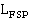), some other attenuation factors are imposed on the radiowave due to medium effects. Consequently, the sum of free-space attenuation (Lfsp) and medium loss (Lm) is defined as basic transmission loss () given by: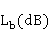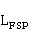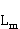(1)

where: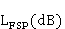= 32.4 + 20log (f) + 20log (d) (2)

where: f is the frequency (MHz) and d is the distance (km). Among others, medium losses include: Atmospheric absorption loss due to gases, vapor, and aerosols; reflection loss, including focusing or defocusing due to curvature of reflecting layer; scattering of radiowave due to irregularities in the atmospheric refractive index or by hydrometeors; diffraction loss due to obstructions; radio precipitation due to rain and snow; temporal climatic effects such as fog and cloud; antenna to medium coupling loss; polarization coupling loss and multipath adverse effects. In most analysis, the value of many of the medium loss components is negligible when compared to others and so they are ignored. In this paper, the medium losses considered include only the ground reflection loss (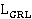) and attenuation due to diffraction (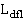). In such case, Blomquist empirical method is used to determine the total path loss as follows [5, 6];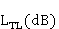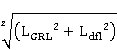(3)

where all the losses are in dB.

In view of the nature of the terrain considered in the case study area, the ITU-R Recommendation P.526-13 method for estimating attenuation due to diffraction over smooth-earth propagation path is use. According to ITU-R Recommendation P.526-13, the standard method for calculating the transmission loss due to diffraction over a smooth-earth is defined as follows , :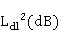= F(X) + G(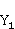) + G(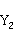)(4)

where: X is the normalised length of the path;(5)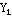and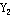are the normalised length antenna heights;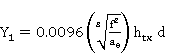(6)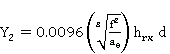(7)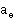is the equivalent Earth radius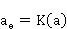a is the actual Earth radius (6370km);

k is the applicable effective earth radius k-factor. The following k-factors are normally applied:

k = 4/3 under the median link planning "standard atmosphere" criteria (50%);

k = 3 under the long term "annual" interference criteria (20%); and

k = 20 under the short term "worst month3" interference criteria (0.01%)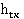is the transmitter antenna height (m);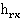is the receiver antenna height (m);

d is the path length (km);

f is the frequency (MHz).

F(X) = 11 + 10 log(X) - 17.6X         (8)

with the height gain term: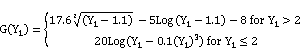(9)(10)

When radio wave propagates over ground, direct ray in addition to ground reflected ray are received. The ground reflection loss in dB is given by the plane earth model as;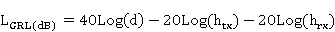Where d is the distance in meters between the transmitter and receiver,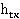is the transmitter antenna height in meters, and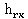is the receiver antenna height in meters.

2.1. Drive Test Measurement Campaign

A handheld Samsung I9500 Galaxy S4 mobile phone was used to take measurement of received signal strength (RSS) from the UMTS 2100 GHz network. The RSS measurements were taken two times along dual lane tarred road in a suburban part of Uyo metropolis. The Samsung I9500 Galaxy S4 has CellMapper Android application installed. The CellMapper captures and displays advanced GSM/CDMA/UMTS/LTE current and neighbouring cells’ low level data and can also record and export the data as comma-separated values (CSV) file. Data captured by the CellMapper comprises the current and neighbouring cells RSS in decibels (dB), the current cells cell ID (CID), local area code (LAC). The RSS along with the respective longitudes and latitudes were recorded at each measurement (receiver) point. In addition, the UMTS base station (transmitter) was located, and its longitude and latitude were recorded.

2.2. Calculation of the Measured Pathloss from the Measured RSS

After the measurements, Haversine formula was used to determine the distance between the mast (transmitter) and of the receiver locations.

The RSS value recorded at each of the receiving point is converted to measured pathloss (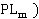in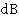by using the formula:=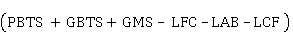– RSS (11)

where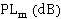is the measured pathloss for each measurement location at a distance d (km) from the base station.

PBTS = Base transceiver station power (dBm),

GBTS = Base transceiver station antenna gain (dBi),

GMS = Mobile station antenna gain (dBi),

LFC = Feeder cable and connector loss (dB),

LAB = Antenna body loss (dB) and

LCF = Combiner and filter loss (dB).

The values of these parameters are given by as: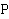BTS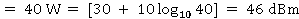; GBTS = 18.15 dBi,

GMS = 0 dBi, LFC = 3 dB, LAB = 3 dB, LCF = 4.7 dB. The measured path loss value in dB obtained for each of the measurement points is recorded in Table 1. The receiver locations, distance, RSS, measured path loss and Okumura-Hata model predicted Pathloss are also given in Table 1.

2.3. Prediction Perf ormance Analysis of the Model

In order to evaluate the prediction performance of the model, the root mean square error (RMSE), prediction accuracy (PA), the absolute minimum prediction error (AMNPE) and the absolute maximum prediction error (AMXPE) are calculated for the models.

Let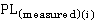be the measured path loss (dB), letbe the predicted path loss (dB) and let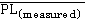be the mean of measured path loss and let n be the number of measured data points. The RMSE is estimated as: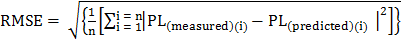(12)

Then, the prediction Accuracy (PA) based on mean absolute percentage error (MAPE) is calculated as: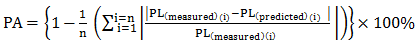(13)

The absolute minimum prediction error (AMNPE) is given for all i as;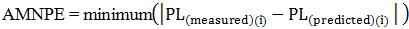(14)

the absolute maximum prediction error (AMXPE) is given for all i as;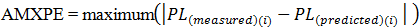(15)

3. Results and Discussion

Table 1 and Table 2 as well as figure 1 show the first and the second drive tests datasets of measured received signal strength (RSSI), measured pathloss and the distance (d) of the measurement points from the transmitter base station.

Table 1. First Dataset Of Measured Received Signal Strength (RSSI), Measured Pathloss and The Distance (d) Of The Measurement Points From The Transmitter Base Station.

 S/N d (km) RSSI (dB) Field Measured Path Loss (dBm) S/N d (km) RSSI (dB) Field Measured Path Loss (dBm) 1 0.1541 -73 126.45 29 0.3823 -75 128.45 2 0.158 -71 124.45 30 0.396 -73 126.45 4 0.1627 -75 128.45 32 0.4242 -73 126.45 5 0.1633 -71 124.45 34 0.452 -71 124.45 7 0.1643 -73 126.45 35 0.4653 -73 126.45 8 0.166 -65 118.45 37 0.4928 -77 130.45 10 0.1683 -69 122.45 38 0.5068 -73 126.45 12 0.1947 -69 122.45 40 0.5332 -73 126.45 13 0.2082 -71 124.45 41 0.5764 -73 126.45 15 0.2345 -75 128.45 43 0.7016 -73 126.45 16 0.2472 -77 130.45 45 0.7308 -73 126.45 18 0.2717 -73 126.45 46 0.7445 -77 130.45 19 0.2827 -71 124.45 48 0.7716 -77 130.45 21 0.2845 -67 120.45 49 0.7848 -77 130.45 23 0.295 -71 124.45 51 0.812 -75 128.45 24 0.311 -73 126.45 52 0.8528 -69 122.45 26 0.338 -71 124.45 54 0.9168 -71 124.45

Table 2. Second Dataset Of Measured Received Signal Strength (RSSI), Measured Pathloss and The Distance (d) Of The Measurement Points From The Transmitter Base Station.

 S/N d (km) RSSI (dB) Field Measured Path Loss (dBm) S/N d (km) RSSI (dB) Field Measured Path Loss (dBm) 1 0.1543 -73 126.45 21 0.389 -73 126.45 2 0.1622 -69 122.45 22 0.4172 -73 126.45 3 0.1627 -73 126.45 23 0.4312 -73 126.45 4 0.1634 -73 126.45 24 0.4588 -71 124.45 5 0.1644 -73 126.45 25 0.4723 -75 128.45 6 0.167 -65 118.45 26 0.4864 -75 128.45 7 0.1749 -69 122.45 27 0.4997 -75 128.45 8 0.1877 -69 122.45 28 0.5137 -73 126.45 9 0.2146 -71 124.45 29 0.5401 -73 126.45 10 0.2281 -75 128.45 30 0.5773 -75 128.45 11 0.2538 -75 128.45 31 0.7092 -75 128.45 12 0.2658 -75 128.45 32 0.7238 -75 128.45 13 0.2772 -71 124.45 33 0.7516 -77 130.45 14 0.2843 -69 122.45 34 0.7652 -77 130.45 15 0.2844 -67 120.45 35 0.7914 -75 128.45 16 0.2875 -71 124.45 36 0.8051 -75 128.45 17 0.3033 -71 124.45 37 0.8675 -65 118.45 18 0.3303 -69 122.45 38 0.9166 -71 124.45 19 0.3463 -73 126.45 39 0.9219 -73 126.45 20 0.3753 -75 128.45 40 0.9275 -73 126.45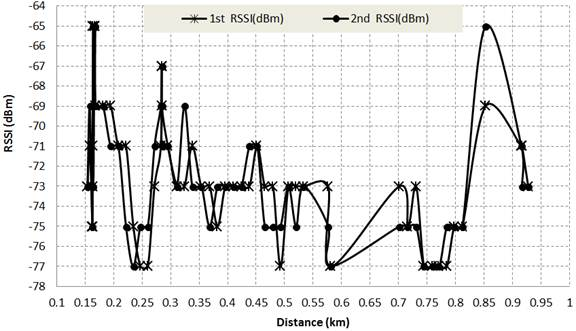Figure 1. Measured RSSI (dBm) Versus Distance (km) For The First and The Second Drive Test Dataset In Table 1 and Table 2.

In the optimization process, the total pathloss in the original Blomquist empirical model is considered as consisting of free space pathloss (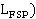and excess pathloss due to ground reflection and diffraction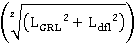. Table 3 and figure 2 show the field measured pathloss (dBm) and the pathloss predicted by the untuned Blomquist model versus distance. The Correlation Coefficient (r) between the prediction residual (error) of the untuned Blomquist model and the excess pathloss due to ground reflection and diffraction is -0.97283. In view of the very strong correction, the optimization process is performed by generating a correction factor which is a function of the excess pathloss that minimizes the root mean square error. The correction factor obtained is given as;

CF = -1.456862685+ 67.8975822 (16)

Hence, the optimized Blomquist empirical model is given as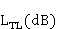= () ++ CF(17)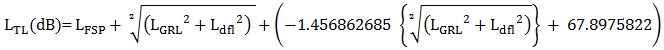(18)

The pathloss predicted by the tuned or optimized Blomquist model versus distance is given in table 3.

Table 3. Measured Pathloss, Pathloss Predicted By Untuned Blomquist Model and Pathloss Predicted By Optimized Blomquist Model Versus Distance.

 S/N d (km) Field Measured Pathloss (dBm) Untuned Blomquist (dBm) Optimized Blomquist (dBm) S/N d (km) Field Measured Pathloss (dBm) Untuned Blomquist (dBm) Optimized Blomquist (dBm) 1 0.1541 126.45 118.4 122.5 19 0.3823 128.45 137.8 125.6 2 0.158 124.45 118.9 122.7 20 0.396 126.45 138.6 125.7 3 0.1627 128.45 119.4 122.7 21 0.4242 126.45 140.2 125.9 4 0.1633 124.45 119.5 122.7 22 0.4384 126.45 141 126 5 0.1643 126.45 119.6 122.8 23 0.4653 126.45 142.4 126.1 6 0.166 118.45 119.8 122.8 24 0.4796 126.45 143.1 126.2 7 0.1683 122.45 120.1 122.9 25 0.5068 126.45 144.3 126.4 8 0.1812 122.45 121.6 123.2 26 0.5202 126.45 145 126.4 9 0.2082 124.45 124.5 123.7 27 0.5764 126.45 147.4 126.7 10 0.2216 124.45 125.8 123.9 28 0.5812 130.45 147.6 126.7 11 0.2472 130.45 128.2 124.3 29 0.7167 128.45 152.6 127.2 12 0.26 130.45 129.2 124.4 30 0.7308 126.45 153 127.2 13 0.2827 124.45 131.1 124.7 31 0.7585 130.45 153.9 127.3 14 0.2844 120.45 131.2 124.7 32 0.7716 130.45 154.3 127.3 15 0.2846 122.45 131.2 124.7 33 0.7979 128.45 155.1 127.4 16 0.295 124.45 132 124.9 34 0.812 128.45 155.6 127.5 17 0.3243 126.45 134.1 125.1 35 0.9156 124.45 158.5 127.8 18 0.338 124.45 135 125.3 36 0.9168 124.45 158.5 127.8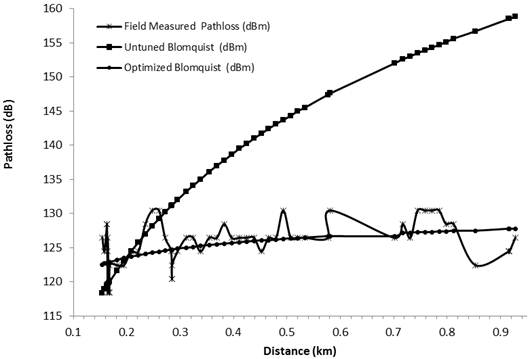Figure 2. Measured Pathloss, Pathloss Predicted By Untuned Blomquist Model and Pathloss Predicted By Optimized Blomquist Model Versus Distance.

The data and pathloss predicted in table 3 are with respect to the training data which is the first drive test field measured data. The second drive test field measured data is used to cross validate the prediction performance of the models. Table 4 and figure 3 show the result of the cross validation process.

Table 4. Cross Validation Field Measured Pathloss, Pathloss Predicted By Untuned Blomquist Model and Pathloss Predicted By Optimized Blomquist Model Versus Distance.

 S/N d (km) Cross Validation Field Measured Pathloss (dBm) Cross Validation Of Untuned Blomquist (dBm) Cross Validation Of Optimised Blomquist (dBm) S/N d (km) Cross Validation Field Measured Pathloss (dBm) Cross Validation Of Untuned Blomquist (dBm) Cross Validation Of Optimised Blomquist (dBm) 1 0.1543 126.45 118.4 123.6 19 0.389 126.45 138.2 126.2 2 0.1622 122.45 119.4 123.8 20 0.4032 126.45 139.1 126.3 3 0.1627 126.45 119.4 123.8 21 0.4312 126.45 140.6 126.4 4 0.1634 126.45 119.5 123.8 22 0.4455 124.45 141.3 126.5 5 0.1644 126.45 119.6 123.8 23 0.4723 128.45 142.7 126.6 6 0.167 118.45 120 123.9 24 0.4864 128.45 143.4 126.7 7 0.1749 122.45 120.9 124 25 0.5137 126.45 144.7 126.8 8 0.1877 122.45 122.3 124.3 26 0.5267 128.45 145.2 126.9 9 0.2146 124.45 125.1 124.7 27 0.5773 128.45 147.4 127 10 0.2281 128.45 126.4 124.9 28 0.5875 130.45 147.8 127.1 11 0.2538 128.45 128.7 125.2 29 0.7238 128.45 152.8 127.4 12 0.2658 128.45 129.7 125.3 30 0.7378 128.45 153.2 127.5 13 0.2843 122.45 131.2 125.5 31 0.7652 130.45 154.1 127.5 14 0.2844 120.45 131.2 125.5 32 0.7781 130.45 154.5 127.6 15 0.2875 124.45 131.4 125.5 33 0.8051 128.45 155.3 127.6 16 0.3033 124.45 132.6 125.6 34 0.8192 128.45 155.8 127.6 17 0.3463 126.45 135.6 126 35 0.9219 126.45 158.6 127.8 18 0.3753 128.45 137.4 126.1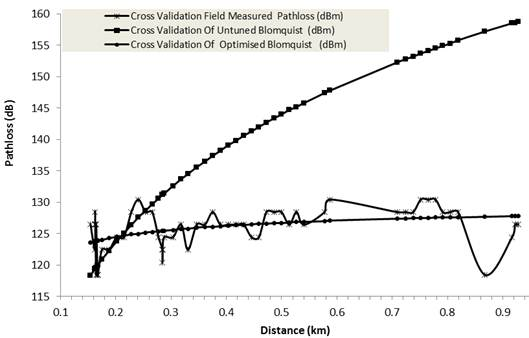Figure 3. Cross Validation Field Measured Pathloss, Pathloss Predicted By Untuned Blomquist Model and Pathloss Predicted By Optimized Blomquist Model Versus Distance.

The prediction performance results in Table 5 show that with the training data (first drive test data), the untuned Blomquist empirical model has RMSE=10.67 dB, Prediction Accuracy = 89.51%, minimum Prediction error = 9.02 dB and maximum Prediction error = -34.29 dB.

Table 5. The Prediction Performance Of The Models.

 Performance With Respect To Training Data Performance With Respect To Cross Validation Data Untuned Blomquist Empirical Model Tuned Or Optimized Blomquist Empirical Model Blomquist Empirical Model Tuned Or Optimized Blomquist Empirical Model RMSE 10.66742 1.749729 10.75249 1.832906 Prediction Accuracy (%) 89.51575 98.30611 89.43392 98.24335 Maximum Prediction Error -34.2904 6.33803 -38.7042 -9.27757 Minimum Prediction Error 9.022973 -4.39509 9.022973 5.432986

With the training data, the optimized Blomquist empirical model has RMSE=1.7497dB, Prediction Accuracy = 98.306%, minimum Prediction error = -4.395 dB and maximum Prediction error = 6.338 dB. Furthermore, with the cross validation data (second drive test data), the optimized Blomquist empirical model has RMSE=1.833 dB, Prediction Accuracy = 98.24%, minimum Prediction error = 5.43 dB and maximum Prediction error = -9.278 dB. The results show that for the given terrain under study, the tuned or optimized Blomquist empirical model can effectively predict the pathloss for the UMTS network signal in the 2100 MHz frequency band.

4. Conclusion

Pathloss prediction for near ground propagation of third generation (3G)-based Universal Mobile Telecommunications System (UMTS) network signal in the 2100 MHz frequency band over a smooth-earth terrain is presented. Attenuation due to diffraction is estimated based on ITU-R Recommendation P.526-13 for diffraction over smooth earth. Also, the total pathloss is determined using the Blomquist empirical model which combined free-space loss, plane-earth loss and the diffraction loss over smooth earth. Two drive tests are conducted for the UMTS 2100 MHz frequency band in suburban area. The results show that for the given terrain under study, the tuned or optimized Blomquist empirical model can effectively predict the pathloss for the UMTS network signal in the 2100 MHz frequency band.

References

1. Isabona, J., Konyeha, C. C., Chinule, C. B., & Isaiah, G. P. (2008). Radio Field Strength Propagation Data and Path Loss Calculation Methods in UMTS Network. Advances in Physics Theories and Applications, 21 (1), 54-68.
2. Isabona J., Konyeha. C. C., Chinule. C. B. and Isaiah G.P. (2013) Radio Field Strength Propagation Data and Pathloss calculation Methods in UMTS Network. Advances in Physics Theories and Applications www.iiste.org Vol. 21, 2013.
3. Obot, A., Simeon, O., and Afolayan J. (2011). Comparative Analysis of Pathloss Prediction Models for Urban Microcellular, Nigerian Journal of Technology Vol. 30, No. 3, pp 50-59.
4. Aziz, J. S., And Hamada, S. T. (2008) Path Profile Analysis of a LOS System Using 3-D Digital Map. The 1 stRegional Conference of Eng. Sci. NUCEJ Spatial ISSUE vol.11,No.1, 2008 pp 28-37.
5. Linnartz, J. P. (2001). Wireless communication: the interactive multimedia CD-ROM. Kluwer Academic Publishers.
6. Linmartz J-P. M. G. (1996) Wireless Communication, The Interactive Multimedia CD-ROM, Baltzer Science Publishers, P.O.Box 37208, 1030 AE Amsterdam, ISSN 1383 4231, Vol. 1 (1996), No. 1.
7. Lodhi, A., Hathi, N., Gkekas, Y., & Nahi, P. (2008, January). Coverage comparison of UMTS networks in 900 and 2100 MHz frequency bands. InWireless, Mobile and Multimedia Networks, 2008. IET International Conference on (pp. 22-25). IET.
8. Chen, X. (2003). U.S. Patent Application No. 10/371,709.
9. Loureiro, A., Gallegos, D., & Caldwell, G. (2011, September). Interference analysis on UMTS-2100 co-existence with GSM-1900. In Vehicular Technology Conference (VTC Fall), 2011 IEEE (pp. 1-4). IEEE.
10. ACMA (2009) Radiocommunications Assignment and Licensing Instructions RALI FX3 (Microwave fixed services frequency coordination). Technical Publication of Australian Communications and Media Authority.Appendix 4: Fixed service propagation modeling. Available at:http://www.acma.gov.au/webwr/radcomm/frequency_planning/frequency_assignment/docs/fx3/fx3apx4.pdf.
11. Recommendation ITU-R P.526-13 (2013), "Propagation by Diffraction," International Telecommunication Union, Geneva, Switzerland, November 2013.

 Contents 1. 2. 2.1. 2.2. 2.3. 3. 4.
Article ToolsAbstractPDF(331K)